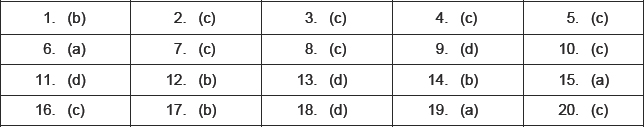Class X Math
MCQ for Arithmetic Progressions
1.   Which of the following is the once of a rhombus?
1.   The first and last term of an A.P. are 1 and 11. If the sum of its terms is 36, then the number of terms will be
(a) 5
(b) 6
(c) 7
(d) 8
2.   If the sum of three consecutive terms of an increasing A.P. is 51 and the product of the first and third of these terms is 273, then the third term is
(a) 13
(b) 9
(c) 21
(d) 17
3.   If 7th and 13th term of an A.P. are 34 and 64 respectively, then its 18th term is
(a) 87
(b) 88
(c) 89
(d) 90
4.   If the first, second and last term of an A.P. are a, b and 2a respectively, its sum is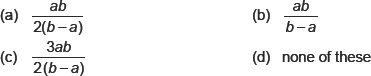5.   If the sum of n terms of an A.P. is then its nth term is
(a) 4n – 3
(b) 3n – 4
(c) 4n + 3
(d) 3n + 4
6.   If 3 times the third term of an A.P. is equal to 5 times the fifth term. Then its 8th term is
(a) 0
(b) 1
(c) 2
(d) 3
7.   In an A.P., am+n + am-n is equal to
(a) 0
(b) 1
(c) 2am
(d) am
8.   If pth term of an A.P.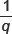is and qth term is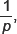then the sum of pq terms is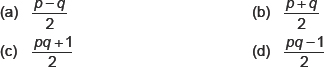9.   If the sum of first n even natural number is equal to k times the sum of first n odd natural number then value of k will be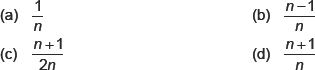10.   If in an A.P., Sn = n2 p, Sm = m2 p and Sr denotes the sum of r terms of the A.P., then is equal to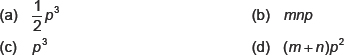11.   The number of terms of an A.P. 3, 7, 11, 15... to be taken so that the sum is 406 is
(a) 5
(b) 10
(c) 12
(d) 14
12.   Sum of n terms of the series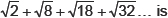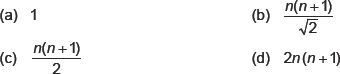13.   The 9th term of an A.P. is 449 and 449th term is 9. The term which is equal to zero is
(a) 508th
(b) 502th
(c) 501th
(d) none of these
14.   If the sum of p terms of an A.P. is q and the sum of q terms is p, then the sum of (p + q) terms will be
(a) p + q
(b) –(p + q)
(c) pq
(d) 0
15.   If are in A.P. then the value of x is
(a) 6
(b) 2
(c) 8
(d) 0
16.   If sum of n terms of an A.P. is then common difference of the A.P. is
(a) 3
(b) 4
(c) 6
(d) 7
17.   Sum of first n natural number is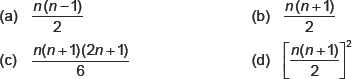18.   If nth terms of the APs 63, 65, 67, ... and 3, 10, 17, ... are equal, then n is
(a) 27
(b) 23
(c) 15
(d) 13
19.   If pth term of an A.P.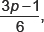is then sum of first n terms of A.P. is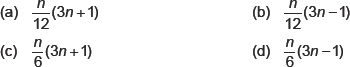20.   Sum of all natural numbers lying between 250 and 1000 which are exactly divisible by 3 is
(a) 157365
(b) 153657
(c) 156375
(d) 155637